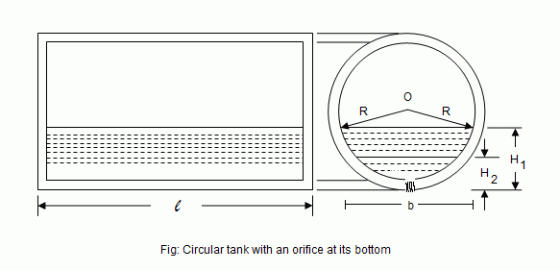•http://facebook.com/
•https://www.google.com/accounts/o8/id
•https://me.yahoo.com

# Circular Horizontal

Time of emptying a circular horizontal tank through an orifice at its bottom

## Overview

Consider a circular horizontal tank, containing liquid and having an orifice at its bottom as shown in figure.Let,
• = Radius of the tank
• = Initial height of the liquid
• = Final height of the liquid
• = Area of the orifice
• = Length of the tank

At some instant, let the height of the liquid be h above the orifice.

We know that the theoretical velocity of the liquid at this instant,

At this instant, let b be the breadth of the liquid in the tank.

Then the surface area of the liquid in the tank,

After a small interval of time dt, let the liquid level fall down by the amount dh.

Therefore volume of the liquid that has passed in time dt,

The value of dh is taken as negative, as its value will decrease with the increase in discharge.

We know that the volume of liquid that has passed through the orifice in time dt,

= Coefficient of discharge Area Theoretical velocity Time

Equating equations (1) and (2)

From the geometry of the tank, we find that,

Substituting this value of in equation (3)

Dividing numerator and denominator by ,

Now the total time (T) required to bring the liquid level from to may be found out by integrating the above equation between the limits to . Therefore

Example:
[metric]
##### Example - Time of emptying a circular horizontal tank
Problem
An orifice is fitted at the bottom of a boiler drum for the purpose of emptying it. The drum is horizontal and half full of water. It is 10m long and 2m in diameter. Find out the time required empty the boiler, if the diameter of the orifice is 150mm. Assume coefficient of discharge as 0.6.
Workings
Given,
• Length of boiler, = 10m
• Diameter of boiler, = 2m
• Diameter of orifice, = 150mm = 0.15m
• = 0.6

We know that the area of the orifice,

So time required to empty the boiler,

This boiler is half full at the time of commencement, and it is to be completely emptied.

Then putting and in the above equation,

Solution
Time to empty the boiler = 8 min 38 s